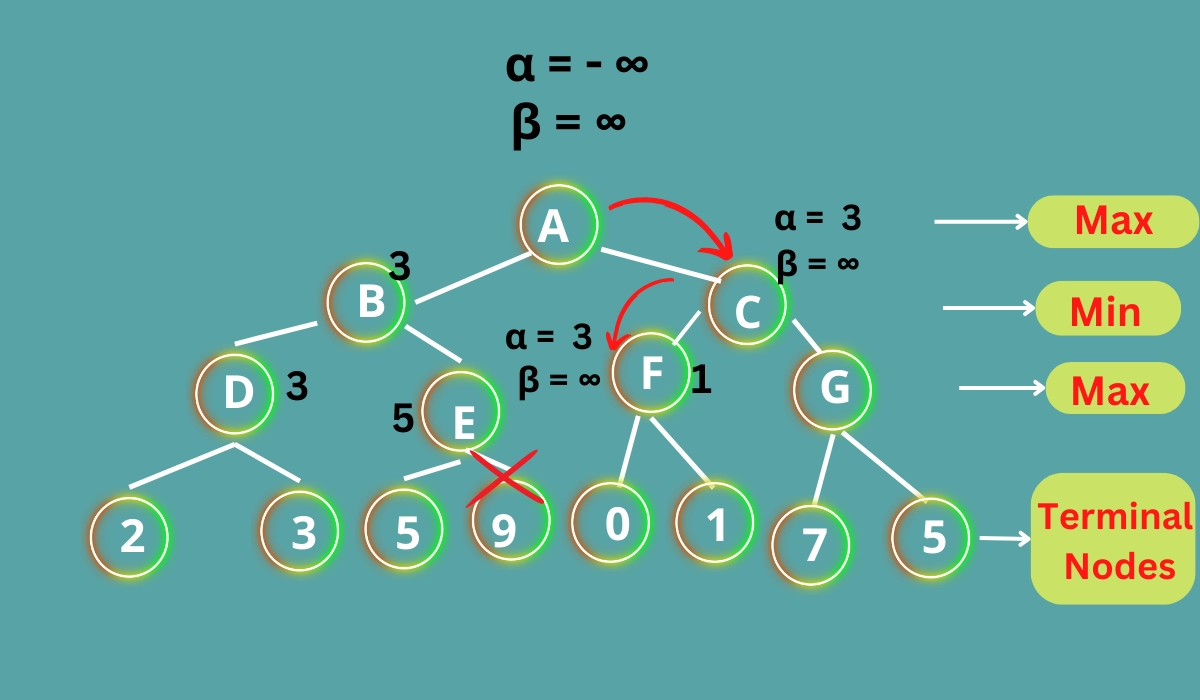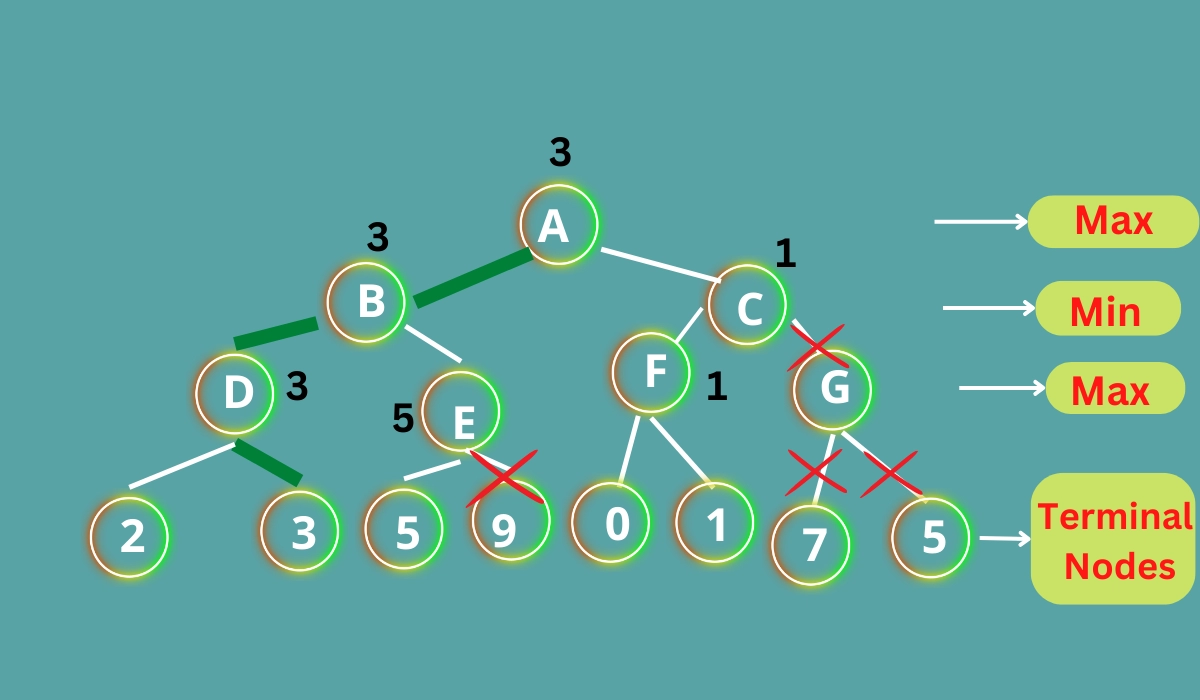New update is available. Click here to update.
Last Updated: Sep 23, 2023
Medium

# Alpha Beta Pruning in Artificial IntelligenceMd Yawar2 upvotes

## Introduction

Artificial intelligence is a broad topic, and there are many algorithms involved in it. One such algorithm is the minimax algorithm. It is a famous backtracking algorithm used in decision-making. Alpha-beta pruning is an optimization technique for the minimax algorithm. Pruning literally means cutting away dead or overgrown branches or stems. In artificial intelligence, pruning means cutting off the useless branches in the decision trees and random forests. Let us learn more about alpha beta pruning in artificial intelligence.

Also, see -  Locally Weighted Regression.

## What is Alpha Beta Pruning?

Alpha beta pruning in Artificial Intelligence is a way of improving the minimax algorithm. In the minimax search method, the number of game states that it must investigate grows exponentially with the depth of the tree. We cannot remove the exponent, but we can reduce it by half. As a result, a technique known as pruning allows us to compute the proper minimax choice without inspecting each node of the game tree. Alpha-beta pruning may be done at any level in a tree, and it can occasionally trim the entire sub-tree and the tree leaves. It is named alpha-beta pruning in artificial intelligence because it involves two parameters, alpha, and Beta.

## Minimax Algorithm

The Minimax algorithm is a backtracking algorithm used in game theory and decision-making. It is used to find the optimal move for a player, assuming that the opponent is also playing optimally. It is commonly used for turn-based two-player games such as chess, checkers, tic-tac-toe, etc.

In minimax, the two players are called minimizer and maximizer. The minimizer tries to get the lowest possible score, while the maximizer attempts to get the maximum possible score. The minimax method determines the best move for MAX, the root node player. The search tree is built by recursively extending all nodes from the root in a depth-first manner until the game ends or the maximum search depth is achieved.

## Condition for Alpha Beta Pruning

The two parameters of alpha beta pruning in artificial intelligence are

Alpha: It is the best highest value choice that we have found in the path of the maximizer. The starting value of alpha is -∞.

Beta: It is the best lowest value choice that we have found in the path of the minimizer. The starting value of beta is +∞.

## Key Points about Alpha Beta Pruning

• Alpha: At each point along the Maximizer path, Alpha is the best option or the highest value we've discovered. – is the initial value for alpha.

• Beta: At every point along the Minimizer route, Beta is the best option or the lowest value we've identified. The value of beta is initially set to +.

• The condition for Alpha-beta Pruning is α >= β.

• Alpha is updated only when it's MAX's time, and Beta can only be updated when it's MIN's turn.

• The MAX player will only update alpha values, whereas the MIN player will only update beta values.

• During the reversal of the tree, node values will be transferred to upper nodes instead of alpha and beta values.

• Only child nodes will get Alpha and Beta values.

## Pseudo-code for Alpha-beta Pruning

Following is the pseudo-code for alpha-beta pruning.

``````function alpha_beta(node, depth, alpha, beta, maximizing_player):
if depth == 0 or node is a terminal node:
return the heuristic value of node

//For max player
if maximizing_player:
v = negative infinity
for each child in node:
v = max(v, alpha_beta(child, depth - 1, alpha, beta, False))
alpha = max(alpha, v)
if beta <= alpha:
break
return v

//For min player
else:
v = infinity
for each child in node:
v = min(v, alpha_beta(child, depth - 1, alpha, beta, True))
beta = min(beta, v)
if beta <= alpha:
break
return v``````

## Working of Alpha Beta Pruning

Let us consider a two-player search tree to understand further how Alpha-beta pruning in artificial intelligence works.

### Step 1

The Max player will start by traveling from node A to node B, where α = - and β = +, and delivering these alpha and beta values to node B, where = - and = + once again, and Node B transmitting the identical value to its offspring D.

### Step 2

As Max's turn at Node D approaches, the value of α will be decided. When the value of α is compared to 2, then 3, the value at node D is max (2, 3) = 3. Hence, the node value is also 3.

### Step 3

The algorithm returns to node B, where the value of β will change since this is a turn of Min. Now β = +∞will be compared to the value of the available subsequent nodes, i.e., min (∞, 3) = 3, resulting in node B now α = -∞, and β = 3. In the next phase, the algorithm will visit the next successor of Node B, Node E, and pass the values of α = -∞ and β = 3.

### Step 4

Max will take over at node E and change alpha's value. The current existing value of alpha will be compared to 5, resulting in max (-∞, 5) = 5, and at node E, where α>=β, the right successor of E will be pruned, and the algorithm will not traverse it, resulting in the value at node E being 5.

### Step 5

We now traverse the tree backward, from node B to node A. At node A, alpha will be converted to the greatest feasible value of 3, as max (-∞, 3)= 3, and β = +∞. These two values will now be passed on to Node C, A's right-hand successor.

At node C, the values and β = +∞ and α =3  will be passed on to node F, and node F will get the identical values.

### Step 6

At node F, the value of α is compared with the left child 0, and max(3,0)= 3. It is then compared with the right child, which is 1, and max(3,1)= 3.### Step 7

Node F sends the node value 1 to node C. The value of Beta is adjusted at C, α = 3 and  β=  +∞, and it is compared to 1, resulting in min (∞, 1) = 1. Now, if α = 3 and β = 1, the condition α>=β is met, the algorithm will prune the next child of C, which is G, rather than calculating the entire sub-tree G.

### Step 8

C now returns the value of 1 to A, with max (3, 1) = 3 being the optimum result for A. The final game tree is shown here, with nodes that have been calculated and nodes that have never been computed. As a result, in this case, the ideal value for the maximizer is 3.## Move Ordering in Alpha Beta Pruning

The sequence in which nodes are inspected determines the efficiency of alpha beta pruning. When it comes to alpha beta pruning in artificial intelligence, move ordering is crucial.

Move ordering are of two types in alpha beta pruning in artificial intelligence:

• Worst Ordering: In some circumstances of alpha beta pruning, the algorithm prunes none of the nodes and behaves like a conventional minimax algorithm. Because of the alpha and beta variables, this takes a long time and produces no valuable findings. In pruning, this is known as the Worst Ordering. In this situation, the optimal move is on the right side of the tree.

• Ideal Ordering: In some circumstances of alpha beta pruning in artificial intelligence, the algorithm prunes a large number of nodes. In pruning, this is referred to as ideal ordering. The optimal move is on the left side of the tree in this situation. We choose DFS Algorithm because it searches the left side of the tree first and then goes deep twice as fast as the minimax method.

## Rules to find Good Ordering

For finding the effective alpha-beta pruning ordering, we have to follow some rules:

• In the tree, sort the nodes such that the better ones are checked first.

• The optimal move is made from the shallowest node.

• We can keep track of the states since there's a chance they'll happen again.

• When deciding on the right step, make use of your subject expertise. For example, in chess, consider the following order: captures first, threats second, forward movements third, backward moves fourth.

## Implementation of Alpha Beta Pruning

``````# Initial values of Alpha and Beta
MAX, MIN = 10000000, -100000000

# Defining the minimax function that returns the optimal value for the current player

def minimax(depth, index, maximizingPlayer,
values, alpha, beta):

# Terminating condition
if depth == 3:
return values[index]

if maximizingPlayer:

optimum = MIN

# Recursion for left and right children
for i in range(0, 2):

val = minimax(depth + 1, index * 2 + i,
False, values, alpha, beta)
optimum = max(optimum, val)
alpha = max(alpha, optimum)

# Alpha Beta Pruning condition
if beta <= alpha:
break

return optimum

else:
optimum = MAX

# Recursion for left and right children
for i in range(0, 2):

val = minimax(depth + 1, index * 2 + i,
True, values, alpha, beta)
optimum = min(optimum, val)
beta = min(beta, optimum)

# Alpha Beta Pruning
if beta <= alpha:
break

return optimum
# Driver Code
if __name__ == "__main__":

values = [5, 7, 12, 6, 3, 18, -9, 4]
print("The value is :", minimax(0, 0, True, values, MIN, MAX))``````

Output:

``The value is 7``

### How does alpha-beta pruning work?

The Minimax algorithm can be optimized using the Alpha Beta Pruning technique. Some of the decision tree's branches are useless and can lead to the same outcome by being ignored. Alpha Beta Pruning removes these useless branches, reducing the exponent time complexity to half at best.

### What are the disadvantages of Alpha Beta Pruning?

The main disadvantage of Alpha Beta pruning is that alpha-beta pruning requires setting a depth limit, as in most cases, it is not feasible to search the entire game tree. The second disadvantage is that it does not solve all the problems associated with the original minimax algorithm.

### What is alpha and beta in alpha-beta pruning method?

Alpha is the best (highest-value) choice we have found so far at any point along the path of Maximizer. The initial value of alpha is -∞. Beta is the best (lowest-value) choice we have found so far at any point along the path of Minimizer.

### Why is it called alpha-beta pruning?

Alpha Beta Pruning uses two parameters called alpha and beta to make the decision of pruning branches. Alpha is used and updated only by the Maximizer and it represents the maximum value found so far. Beta is used and updated only by the Minimizer and it represents the minimum value found.

### What are the properties of alpha-beta pruning?

It eliminates all the paths that are not optimal for the result. It uses the Depth-First Search approach to explore the nodes, and only after exploring all children of a node, the result is moved up.

## Conclusion

This blog talked about Alpha beta pruning in artificial intelligence. We learned about the minimax algorithm and how it can be modified or improved using alpha beta pruning.